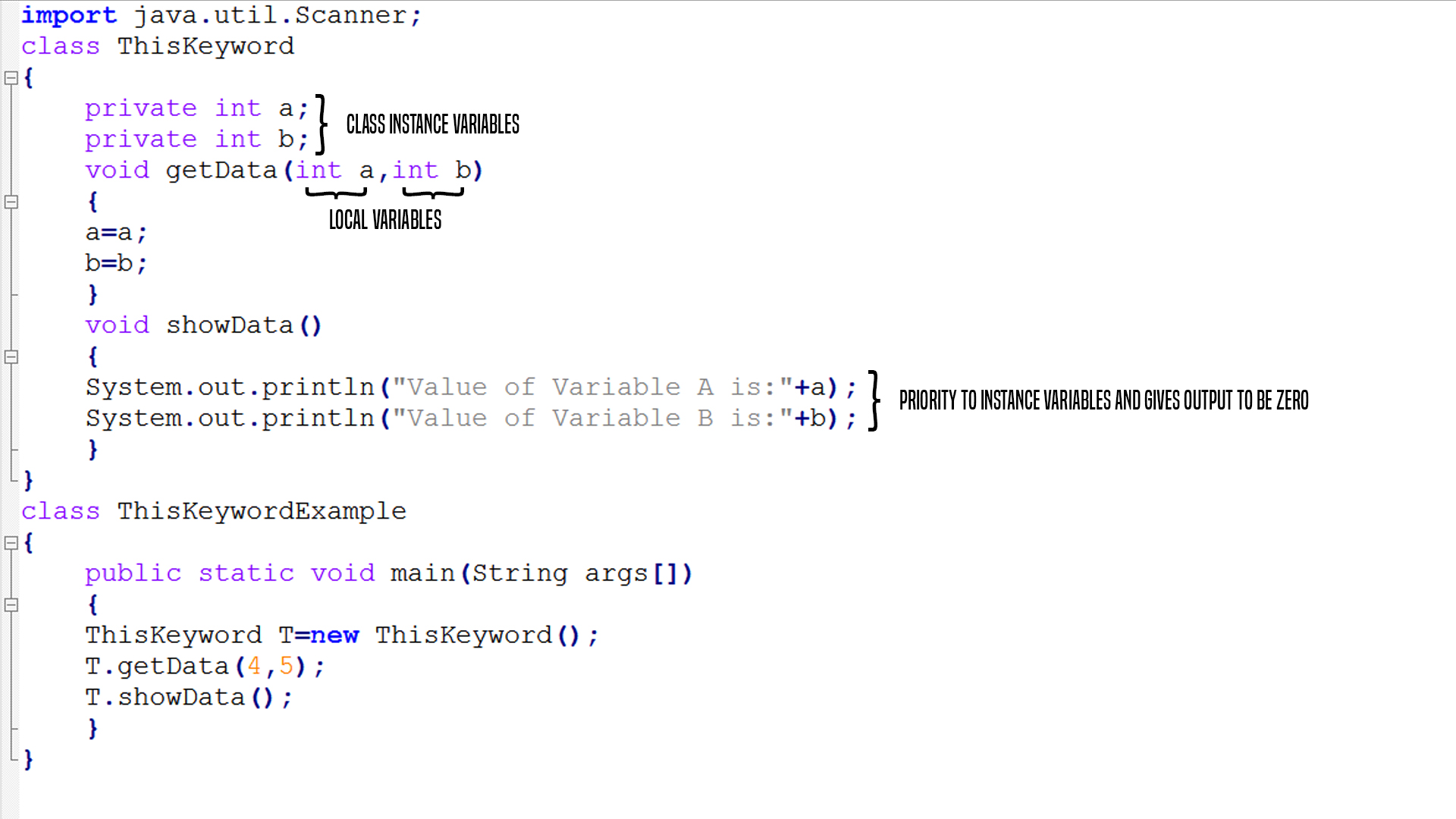# Java - this reference

Learn: this keyword in Java, in this article we will learn about this Reference, how it can be used when both the Member Name and Parameter name of any method are same.
Submitted by Mayank Singh, on June 17, 2017

## this Object

this is an Object which holds the Reference of another Object which invokes the member function.

Consider the program:

```import java.util.Scanner;
class ThisKeyword
{
private int a;
private int b;
void getData(inta,int b)
{
a=a;
b=b;
}
void showData()
{
System.out.println("Value of Variable A is:"+a);
System.out.println("Value of Variable B is:"+b);
}
}
class ThisKeywordExample
{
public static void main(String args[])
{
ThisKeyword T=new ThisKeyword();
T.getData(4,5);
T.showData();
}
}
```

Output

```Value of Variable A is:0
Value of Variable B is:0
```

Explanation of the Output.the body of getData() method the compiler is confused whether it should give priority to Instance Variables or to Local Variables and that is why in the showData() method , Compiler give priority to the Instance variables and give output equal to zero.

We can avoid this by using this reference variable in the getData() method as follows:

```this.a=a;
this.b=b;
```

When the object T invokes the getData() method, this reference is replaced by the reference of the object T so that :

```T.a=a;
T.b=b;
```

Thus, T.a is the instance variables and a is the local variable as defined in the getData() method's parameter.

Consider the program:

```import java.util.Scanner;
class ThisKeyword
{
private int a;
private int b;
void getData(int a,int b)
{
this.a=a;
this.b=b;
}
void showData()
{
System.out.println("Value of Variable A is:"+a);
System.out.println("Value of Variable B is:"+b);
}
}
class ThisKeywordExample
{
public static void main(String args[])
{
ThisKeyword T=new ThisKeyword();
T.getData(4,5);
T.showData();
}
}
```

Output

```Value of Variable A is:4
Value of Variable B is:5
```

Let us consider another example Where we will be using this keyword differently.

Our main aim in the following program is to find out who is Elder in Age among two Persons, we will be implementing this program with the help of this keyword.

```import java.util.Scanner;

class Person
{
private String name;
private int age;
Scanner KB=new Scanner(System.in);

void getPerson()
{
System.out.println("Enter the Name of the Person:");
name=KB.nextLine();
System.out.println("Enter the Age of the Person:");
age=KB.nextInt();
}

void putPerson()
{
System.out.println("Name: "+name);
System.out.println("Age: "+age);
}

Person WhoIsElder(Person P)
{
if(P.age>age)
{
return P;
}
else if(P.age==age)
{
return null;
}
else
{
return this;
}
}
}

class ElderPerson
{
public static void main(String args[])
{
Person P1=new Person();
P1.getPerson();
Person P2=new Person();
P2.getPerson();
Person ReferenceHolder;
ReferenceHolder=P1.WhoIsElder(P2);
if(ReferenceHolder==null)
{
System.out.println("Both the Persons have Same Age");
}
else
{
System.out.println("Elder Person :");
ReferenceHolder.putPerson();
}
}
}
```

Output

```Enter the Name of the Person:
Mayank Singh
Enter the Age of the Person:
18
Enter the Name of the Person:
Amit Shukla
Enter the Age of the Person:
17
Elder Person :
Name :Mayank Singh
Age :18
```

If both the Person have had same Age, Output:

```Enter the Name of the Person:
Mayank Singh
Enter the Age of the Person:
18
Enter the Name of the Person:
Amit Shukla
Enter the Age of the Person:
18
Both the Person have Same Age
```